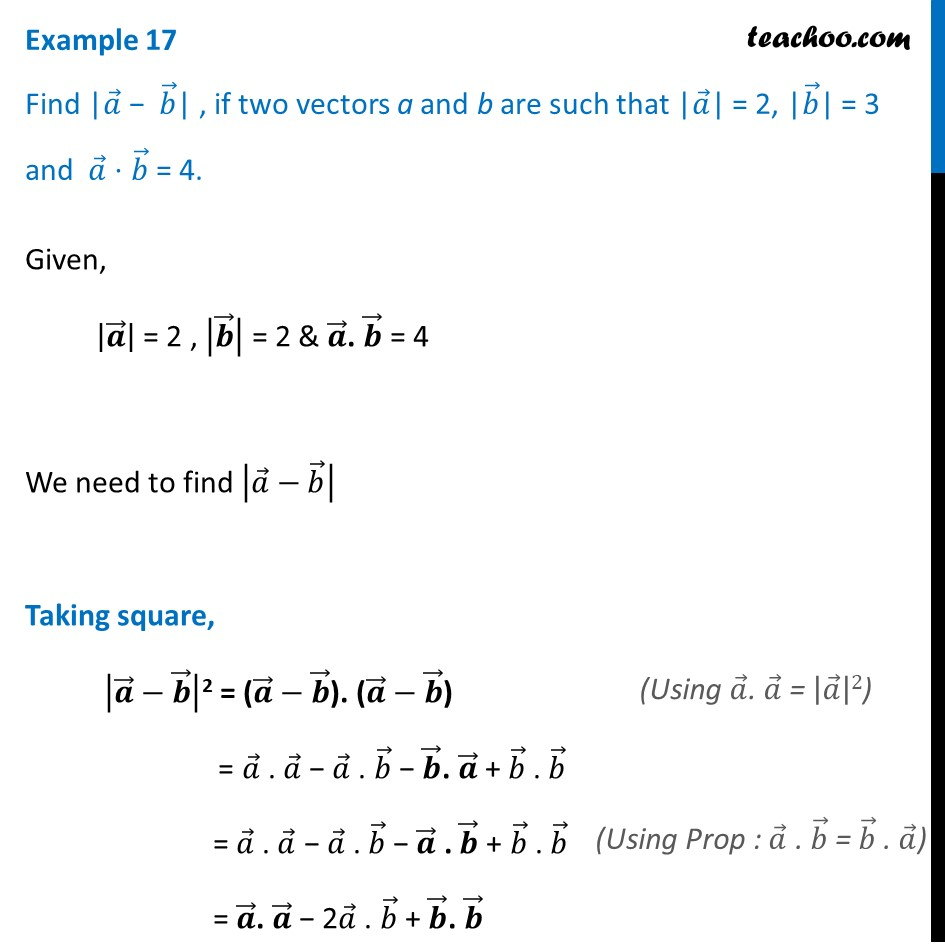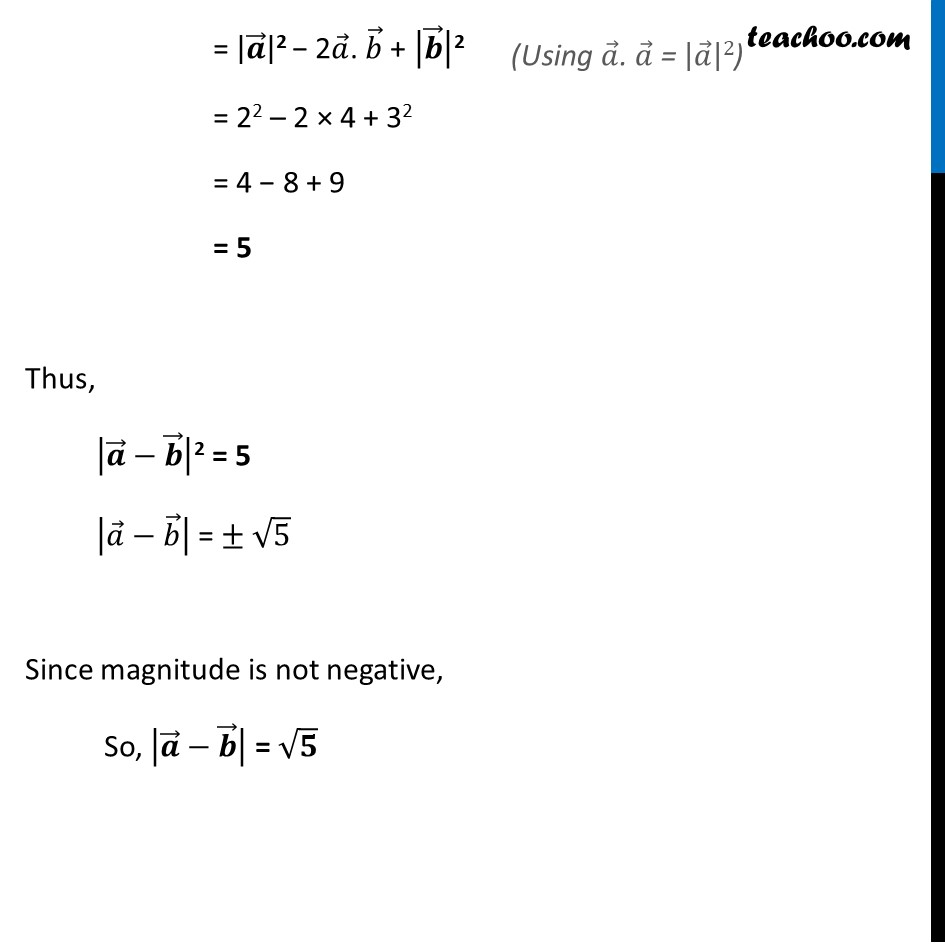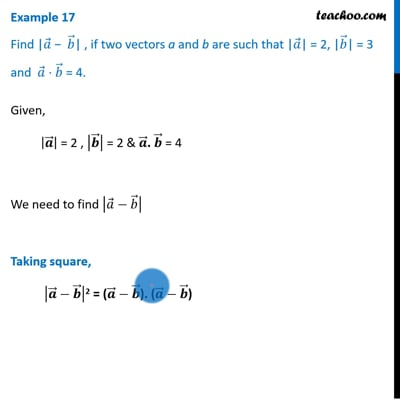Examples

Chapter 10 Class 12 Vector Algebra
Serial order wiseThis video is only available for Teachoo black users

Solve all your doubts with Teachoo Black (new monthly pack available now!)

### Transcript

Example 17 Find |𝑎 ⃗ − 𝑏 ⃗| , if two vectors a and b are such that |𝑎 ⃗| = 2, |𝑏 ⃗| = 3 and 𝑎 ⃗ ⋅ 𝑏 ⃗ = 4. Given, |𝒂 ⃗ | = 2 , |𝒃 ⃗ | = 2 & 𝒂 ⃗. 𝒃 ⃗ = 4 We need to find |𝑎 ⃗−𝑏 ⃗ | Taking square, |𝒂 ⃗−𝒃 ⃗ |2 = (𝒂 ⃗−𝒃 ⃗). (𝒂 ⃗−𝒃 ⃗) = 𝑎 ⃗ . 𝑎 ⃗ − 𝑎 ⃗ . 𝑏 ⃗ − 𝒃 ⃗. 𝒂 ⃗ + 𝑏 ⃗ . 𝑏 ⃗ = 𝑎 ⃗ . 𝑎 ⃗ − 𝑎 ⃗ . 𝑏 ⃗ − 𝒂 ⃗ . 𝒃 ⃗ + 𝑏 ⃗ . 𝑏 ⃗ = 𝒂 ⃗. 𝒂 ⃗ − 2𝑎 ⃗ . 𝑏 ⃗ + 𝒃 ⃗. 𝒃 ⃗ = |𝒂 ⃗ |2 − 2𝑎 ⃗. 𝑏 ⃗ + |𝒃 ⃗ |2 = 22 – 2 × 4 + 32 = 4 − 8 + 9 = 5 Thus, |𝒂 ⃗−𝒃 ⃗ |2 = 5 |𝑎 ⃗−𝑏 ⃗ | = ± √5 Since magnitude is not negative, So, |𝒂 ⃗−𝒃 ⃗ | = √𝟓